# 8.1 K邻近分类法KNN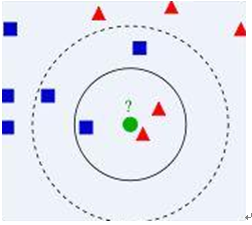1.算距离：给定测试对象，计算它与训练集中的每个对象的距离；
2.找邻居：圈定距离最近的k个训练对象，作为测试对象的近邻；
3. 做分类：根据这k个近邻归属的主要类别，来对测试对象分类。

class KnnClassifier(object):
def __init__(self,labels,samples):
""" 使用训练数据初始化分类器 """
self.labels = labels
self.samples = samples
def classify(self,point,k=3):
""" 在训练数据上采用 k 近邻分类，并返回标记 """

# 计算所有训练数据点的距离
dist = array([L2dist(point,s) for s in self.samples])

# 对它们进行排序
ndx = dist.argsort()

# 用字典存储 k 近邻
for i in range(k):
label = self.labels[ndx[i]]

def L2dist(p1,p2):
return sqrt( sum( (p1-p2)**2) )


## 8.1.1 一个简单的二维示例

from numpy.random import randn
import pickle
# 创建二维样本数据
n = 200
# 两个正态分布数据集
class_1 = 0.6 * randn(n,2)
class_2 = 1.2 * randn(n,2) + array([5,1])
labels = hstack((ones(n),-ones(n)))
# 用 Pickle 模块保存
with open('points_normal.pkl', 'w') as f:
pickle.dump(class_1,f)
pickle.dump(class_2,f)
pickle.dump(labels,f)
# 正态分布，并使数据成环绕状分布
class_1 = 0.6 * randn(n,2)
r = 0.8 * randn(n,1) + 5
angle = 2*pi * randn(n,1)
class_2 = hstack((r*cos(angle),r*sin(angle)))
labels = hstack((ones(n),-ones(n)))
# 用 Pickle 保存
with open('points_ring.pkl', 'w') as f:
pickle.dump(class_1,f)
pickle.dump(class_2,f)
pickle.dump(labels,f)


# -*- coding: utf-8 -*-
import pickle
from PCV.classifiers import knn
from PCV.tools import imtools
from pylab import *
from numpy import *

with open('points_normal_t.pkl', 'r') as f:
model = knn.KnnClassifier(labels,vstack((class_1,class_2)))

with open('points_normal_test.pkl', 'r') as f:

print model.classify(class_1)

# 定义绘图函数
def classify(x,y,model=model):
return array([model.classify([xx,yy]) for (xx,yy) in zip(x,y)])
# 绘制分类边界
imtools.plot_2D_boundary([-6,6,-6,6],[class_1,class_2],classify,[1,-1])
show()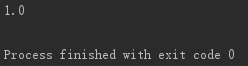def plot_2D_boundary(plot_range,points,decisionfcn,labels,values=):
"""Plot_range 为（xmin，xmax，ymin，ymax），points 是类数据点列表，
decisionfcn 是评估函数，labels 是函数 decidionfcn 关于每个类返回的标记列表 """
clist = ['b','r','g','k','m','y'] # 不同的类用不同的颜色标识

# 在一个网格上进行评估，并画出决策函数的边界
x = arange(plot_range,plot_range,.1)
y = arange(plot_range,plot_range,.1)
xx,yy = meshgrid(x,y)
xxx,yyy = xx.flatten(),yy.flatten() # 网格中的 x，y 坐标点列表
zz = array(decisionfcn(xxx,yyy))
zz = zz.reshape(xx.shape)
# 以 values 画出边界
contour(xx,yy,zz,values)
# 对于每类，用 * 画出分类正确的点，用 o 画出分类不正确的点
for i in range(len(points)):
d = decisionfcn(points[i][:,0],points[i][:,1])
correct_ndx = labels[i]==d
incorrect_ndx = labels[i]!=d
plot(points[i][correct_ndx,0],points[i][correct_ndx,1],'*',color=clist[i])
plot(points[i][incorrect_ndx,0],points[i][incorrect_ndx,1],'o',color=clist[i])
axis('equal')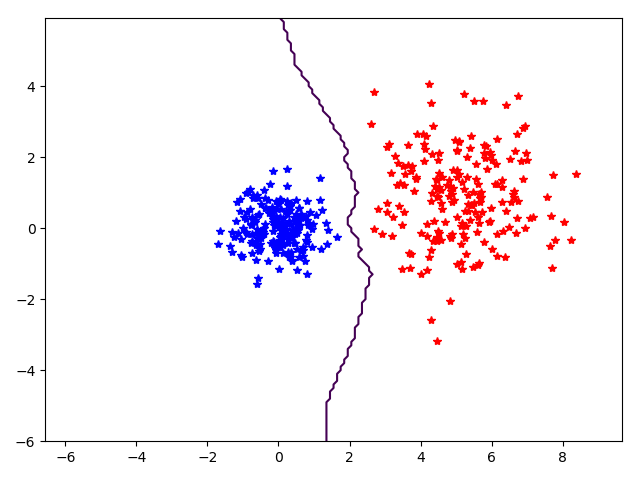## 8.1.2 用稠密的SIFT作为图像特征

import sift
def process_image_dsift(imagename,resultname,size=20,steps=10,
force_orientation=False,resize=None):
""" 用密集采样的 SIFT 描述子处理一幅图像，并将结果保存在一个文件中。可选的输入：
特征的大小 size，位置之间的步长 steps，是否强迫计算描述子的方位 force_orientation
（False 表示所有的方位都是朝上的），用于调整图像大小的元组 """
im = Image.open(imagename).convert('L')
if resize!=None:
im = im.resize(resize)
m,n = im.size
if imagename[-3:] != 'pgm':
# 创建一个 pgm 文件
im.save('tmp.pgm')
imagename = 'tmp.pgm'
# 创建帧，并保存到临时文件
scale = size/3.0
x,y = meshgrid(range(steps,m,steps),range(steps,n,steps))
xx,yy = x.flatten(),y.flatten()
frame = array([xx,yy,scale*ones(xx.shape),zeros(xx.shape)])
savetxt('tmp.frame',frame.T,fmt='%03.3f')
if force_orientation:
cmmd = str("sift "+imagename+" --output="+resultname+
else:
cmmd = str("sift "+imagename+" --output="+resultname+
os.system(cmmd)
print 'processed', imagename, 'to', resultname


from PCV.localdescriptors import dsift,sift
from numpy import *
from PIL import Image
from pylab import *

dsift.process_image_dsift('shangdalou.jpg','shangdalou.sift',90,40,True)
im = array(Image.open('shangdalou.jpg'))
sift.plot_features(im,l,True)
show()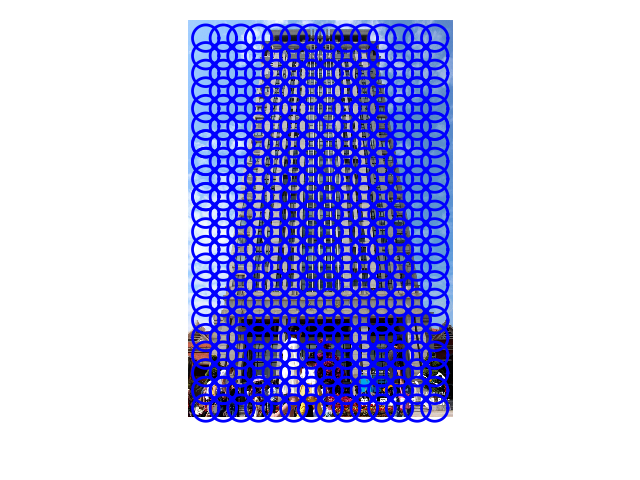## 8.1.3 图像分类：手势识别

# -*- coding: utf-8 -*-
import os
from PCV.localdescriptors import sift, dsift
from pylab import *
from PIL import Image

imlist=['D:/uniform/train/C-uniform01.ppm', 'D:/uniform/train/B-uniform01.ppm',
'D:/uniform/train/A-uniform01.ppm', 'D:/uniform/train/Five-uniform01.ppm',
'D:/uniform/train/Point-uniform01.ppm', 'D:/uniform/train/V-uniform01.ppm']

figure()
for i, im in enumerate(imlist):
print (im)
dsift.process_image_dsift(im,im[:-3]+'dsift',50,20,True)
dirpath, filename=os.path.split(im)
im = array(Image.open(im))
#显示手势含义title
titlename=filename[:-14]
subplot(2,3,i+1)
sift.plot_features(im,l,True)
title(titlename)
show()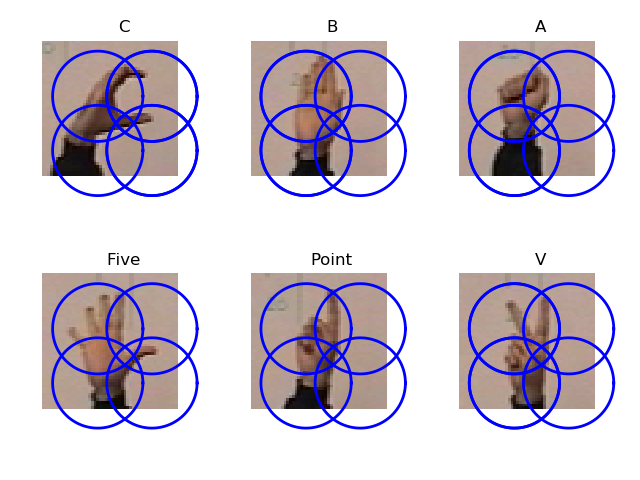# -*- coding: utf-8 -*-
from PCV.localdescriptors import dsift
import os
from PCV.localdescriptors import sift
from pylab import *
from PCV.classifiers import knn

def get_imagelist(path):
""" Returns a list of filenames for
all jpg images in a directory. """

return [os.path.join(path,f) for f in os.listdir(path) if f.endswith('.ppm')]

# create list of all files ending in .dsift
featlist = [os.path.join(path,f) for f in os.listdir(path) if f.endswith('.dsift')]
features = []
for featfile in featlist:
features.append(d.flatten())
features = array(features)
# create labels
labels = [featfile.split('/')[-1] for featfile in featlist]
return features,array(labels)

def print_confusion(res,labels,classnames):
n = len(classnames)
# confusion matrix
class_ind = dict([(classnames[i],i) for i in range(n)])
confuse = zeros((n,n))
for i in range(len(test_labels)):
confuse[class_ind[res[i]],class_ind[test_labels[i]]] += 1
print 'Confusion matrix for'
print classnames
print confuse

filelist_train = get_imagelist('D:\\uniform\\train')
filelist_test = get_imagelist('D:\\uniform\\test')
imlist=filelist_train+filelist_test

# process images at fixed size (50,50)
for filename in imlist:
featfile = filename[:-3]+'dsift'
dsift.process_image_dsift(filename,featfile,10,5,resize=(50,50))

classnames = unique(labels)

# test kNN
k = 1
knn_classifier = knn.KnnClassifier(labels,features)
res = array([knn_classifier.classify(test_features[i],k) for i in
range(len(test_labels))])
# accuracy
acc = sum(1.0*(res==test_labels)) / len(test_labels)
print 'Accuracy:', acc

print_confusion(res,test_labels,classnames)


Accuracy: 0.811518324607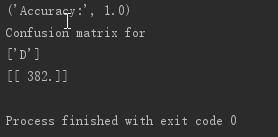# 8.2 贝叶斯分类器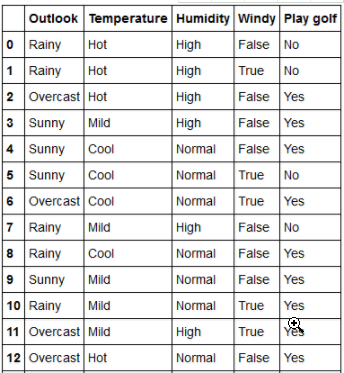1.特征矩阵包含数据集中所有的向量（行），每个向量是由依赖特征组成的。在上面的数据集中，特征就是“天气”，“温度”，“湿度”还有“刮风”。
2.响应向量包含的是特征矩阵每一行的类变量（预测或者输出）值。在上述的数据集中，类变量名为“Play golf”。

1，我们假设没有特征对是相互依赖的。温度热不热跟湿度没有任何关系，天气是否下雨也不影响是否刮风。因此，这就是假设特征相互独立。
2，其次，每个特征都有相同的权重（或者是重要性）。例如，只知道温度和湿度是不能准确地推断出结果的。任何属性都与结果是有关系的，并且影响程度是相同的。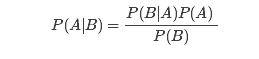1，基本上，只要我们给出了事件B为真，那么就能算出事件A发生的概率，事件B也被称为证据。
2，P(A)是事件A的先验（先验概率，例如，在证据之前发生的概率）。证据是一个未知事件的一个属性值（在这里就是事件B）。
3，P(A|B)是B的后验概率，例如在证据之后发生的概率。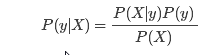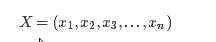X = (Rainy, Hot, High, False)
y = No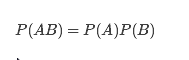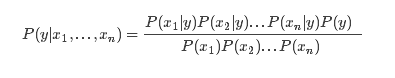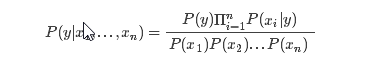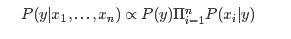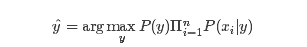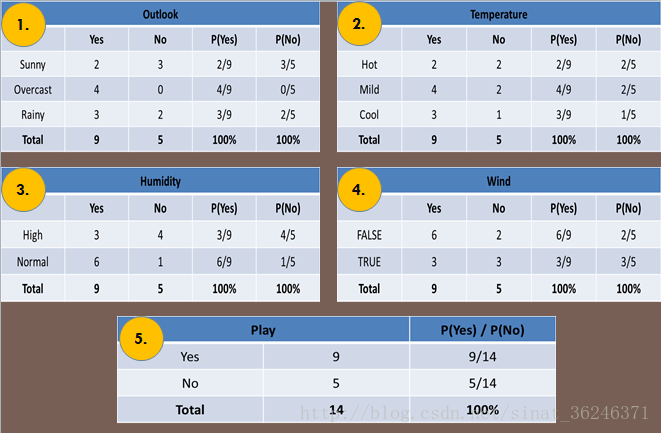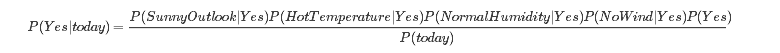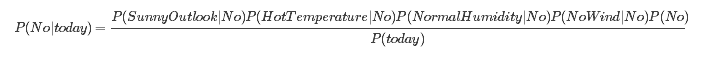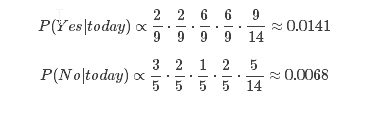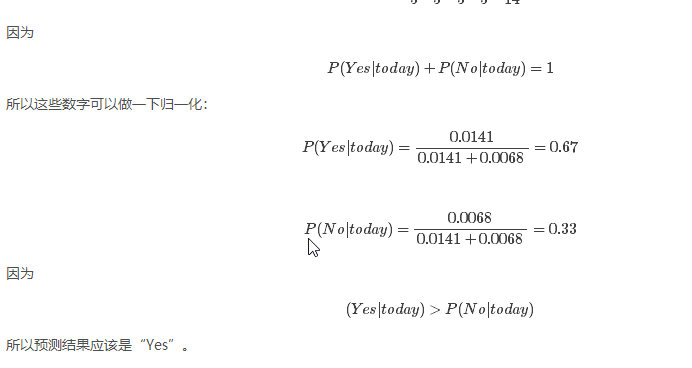Classifier 类添加到文件 bayes.py 中：

class BayesClassifier(object):
def __init__(self):
""" 使用训练数据初始化分类器 """
self.labels = [] # 类标签
self.mean = [] # 类均值
self.var = [] # 类方差
self.n = 0 # 类别数
def train(self,data,labels=None):
""" 在数据 data（n×dim 的数组列表）上训练，标记 labels 是可选的，默认为 0…n-1 """
if labels==None:
labels = range(len(data))
self.labels = labels
self.n = len(labels)
for c in data:
self.mean.append(mean(c,axis=0))
self.var.append(var(c,axis=0))
def classify(self,points):
""" 通过计算得出的每一类的概率对数据点进行分类，并返回最可能的标记 """
# 计算每一类的概率
est_prob = array([gauss(m,v,points) for m,v in zip(self.mean,self.var)])
# 获取具有最高概率的索引，该索引会给出类标签
ndx = est_prob.argmax(axis=0)
est_labels = array([self.labels[n] for n in ndx])
return est_labels, est_prob


（每个类对应一个特征数组），并计算每个特征数组的均值和协方差。classify() 方

def gauss(m,v,x):
""" 用独立均值 m 和方差 v 评估 d 维高斯分布 """
if len(x.shape)==1:
n,d = 1,x.shape
else:
n,d = x.shape
# 协方差矩阵，减去均值
S = diag(1/v)
x = x-m
# 概率的乘积
y = exp(-0.5*diag(dot(x,dot(S,x.T))))
# 归一化并返回
return y * (2*pi)**(-d/2.0) / ( sqrt(prod(v)) + 1e-6)


import pickle
from PCV.classifiers import bayes
from PCV.tools import imtools
from pylab import *

with open('points_normal_t.pkl', 'r') as f:

bc = bayes.BayesClassifier()
bc.train([class_1,class_2],[1,-1])

with open('points_normal_test.pkl', 'r') as f:

print bc.classify(class_1[:10])

def classify(x, y, bc=bc):
points = vstack((x, y))
return bc.classify(points.T)

imtools.plot_2D_boundary([-6, 6, -6, 6], [class_1, class_2], classify, [1, -1])
show()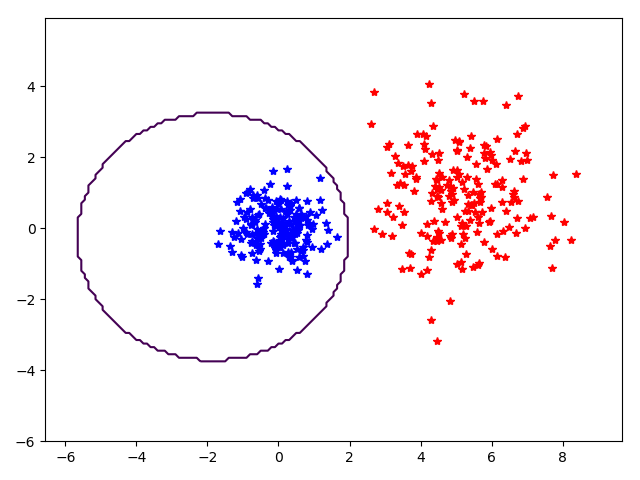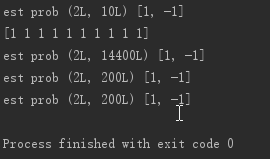# 8.3 支持向量机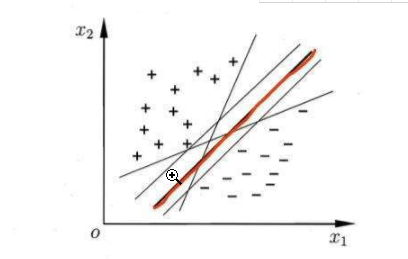，因为该划分超平面对训练样本局部扰动的“容忍”性最好，例如，由于训练集的局限性或者噪声的因素，训练集外的样本可能比图中的训练样本更接近两个类的分隔界，这将使许多划分超平面出现错误。而红色超平面的影响最小，简言之，这个划分超平面所产生的结果是鲁棒性的。

​ 那什么是线性可分呢？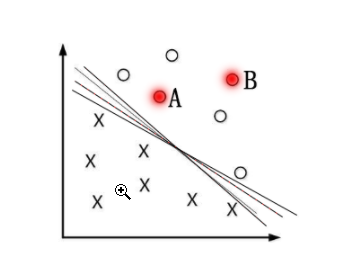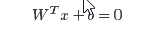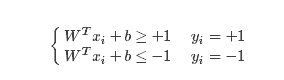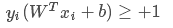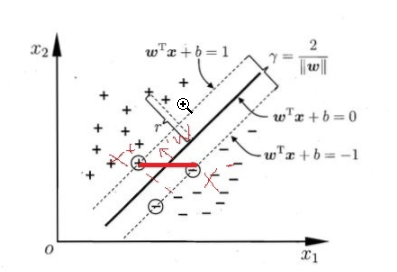​ 关于间隔的计算：它就等于两个异类支持向量的差在 W方向上的投影 ，W方向是指图所示实线的法线方向。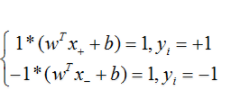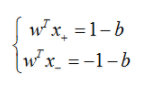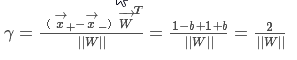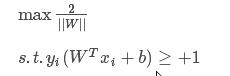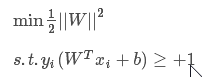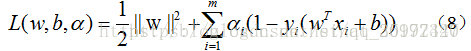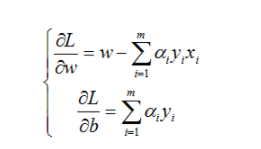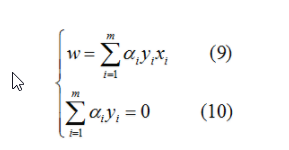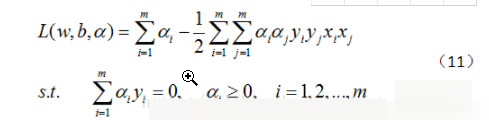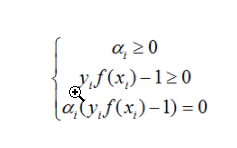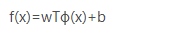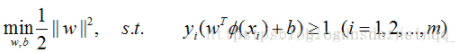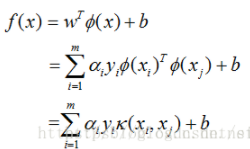（数学推导过程摘自https://blog.csdn.net/qq_35992440/article/details/80987664）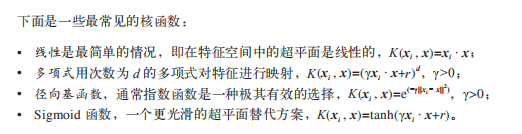## 使用LibSVM

https://www.lfd.uci.edu/~gohlke/pythonlibs/#libsvm

LibSVM 是最好的、使用最广泛的 SVM 实现工具包。LibSVM 为 Python 提供了

import pickle
from svmutil import *
import imtools
# 用 Pickle 载入二维样本点
with open('points_normal.pkl', 'r') as f:
# 转换成列表，便于使用 libSVM
class_1 = map(list,class_1)
class_2 = map(list,class_2)
labels = list(labels)
samples = class_1+class_2 # 连接两个列表
# 创建 SVM
prob = svm_problem(labels,samples)
param = svm_parameter('-t 2')
# 在数据上训练 SVM
m = svm_train(prob,param)
# 在训练数据上分类效果如何？
res = svm_predict(labels,samples,m)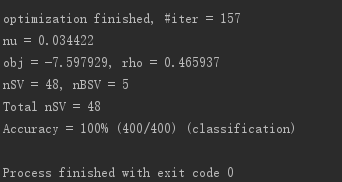# 用 Pickle 模块载入测试数据
with open('points_normal_test.pkl', 'r') as f:
# 转换成列表，便于使用 LibSVM
class_1 = map(list,class_1)
class_2 = map(list,class_2)
# 定义绘图函数
def predict(x,y,model=m):
return array(svm_predict(*len(x),zip(x,y),model))
# 绘制分类边界
imtools.plot_2D_boundary([-6,6,-6,6],[array(class_1),array(class_2)],predict,[-1,1])
show()


(*len(x)) 列表来代替标记列表。只要代替的标记列表长度正确，你可以使用任意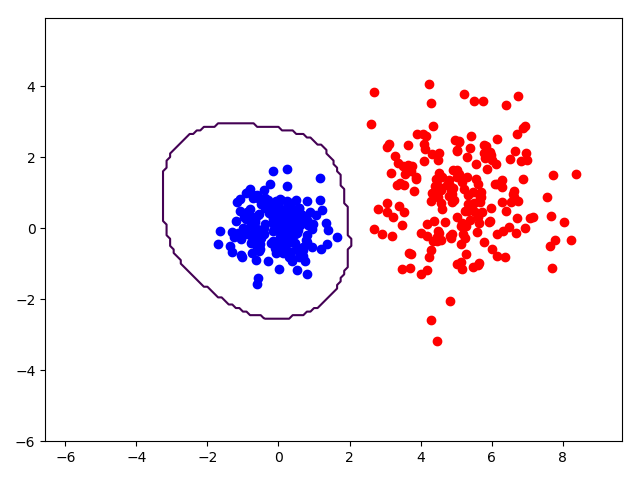©️2019 CSDN 皮肤主题: 大白 设计师: CSDN官方博客